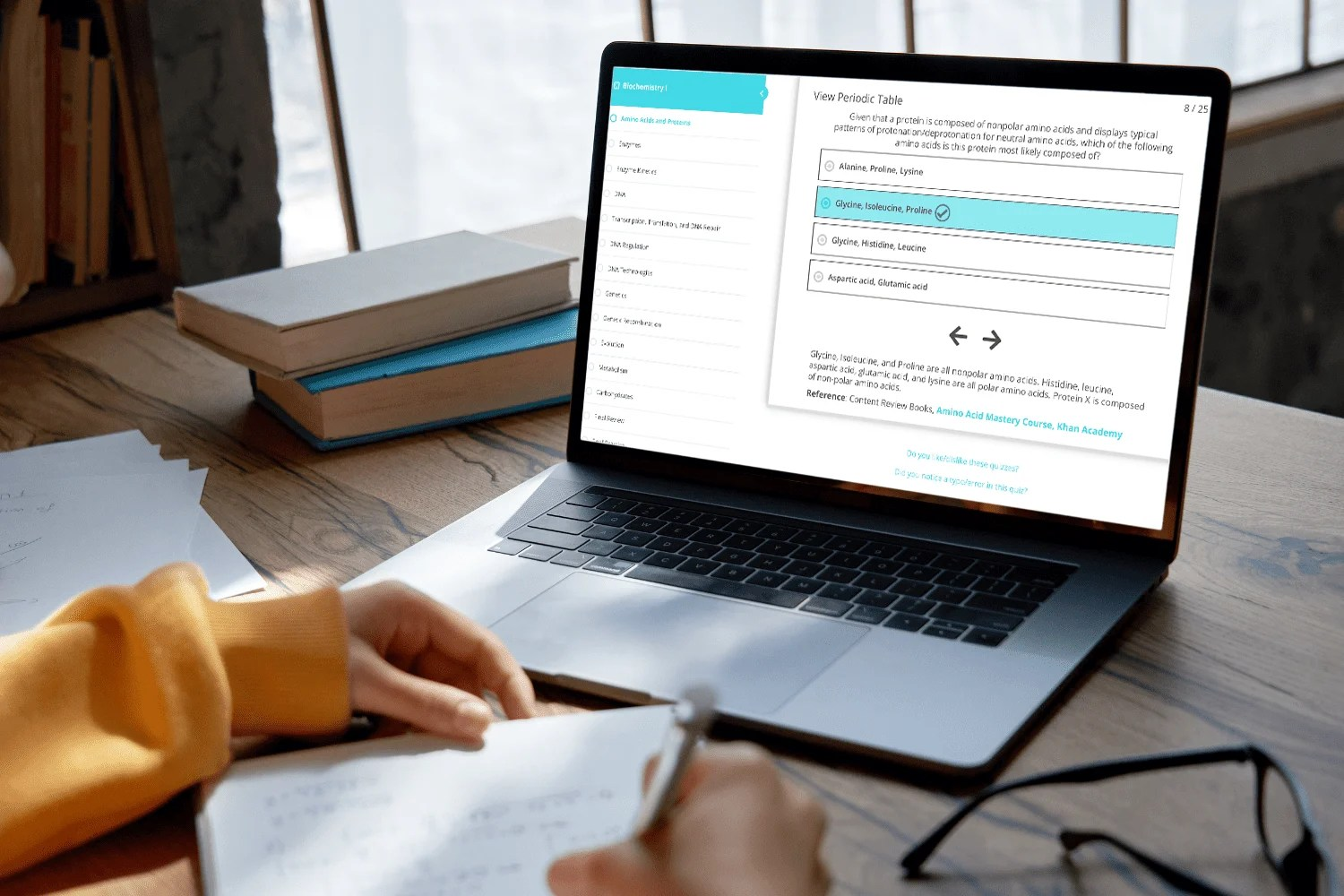Select Page

# Free Practice Quizzes

As part of our free eCourse, you will have access to 120 quizzes integrated into each lesson of the eCourse for a total of 360 questions designed to test your understanding of the content covered in that lesson.

Our End-of-Lesson Quizzes are written in the same style as those written by the AAMC, the writers of the actual MCAT. Each quiz question includes answer explanations, worked math solutions, pictures, and diagrams. As an added bonus, you can review and retake quizes anytime.# Try an End-of-Lesson Quiz!

0%

Lesson 12: Thermodynamics Part II

Thermodynamics Part II

View Periodic Table1 / 3

A researcher carries out the following reaction at two different conditions to form methane gas:

CS(g) + 4H(g) ---> CH(g) + 2HS(g)

In Condition 1, the reaction is performed at 80 K and 0.025 atm, and the experimenter calculates the entropy of methane to be 200 J/molᐧK. In Condition 2, methane forms at 150 K and 0.005 atm. The entropy of methane in this condition is calculated to be 188 J/molᐧK. Did the experimenter make an error in his calculations, why or why not?

View Periodic Table2 / 3

The use of gas turbine engines is the most common way for airplanes to generate thrust. The gas within these engines undergoes a series of thermodynamic processes by which it eventually returns to its original state. Which of the following must be true of the gas turbine engine system once the cycle is complete?

I. The internal energy will have increased.

II. The work done on the system will be equal to the heat added to the system.

III. The pressure and volume will not have changed.

View Periodic Table3 / 3

A physicist conducts an experiment in which he observes a closed system containing Ne and H₂ gas particles at two different points in time. He compares the state of the system at the beginning of the experiment to the state of the system at t = 2 hours. What must be true of the system 2 hours after the start of the experiment?

The average score is 48%

0%

## 120 Lessons, 120 Quizzes

#### Double Check Understanding

After working through the content in each lesson of the free eCourse, students can take the corresponding End-of-Lesson Mastery Quiz to confirm that they learned the key concepts within each lesson.

#### Save Time

It is extremely time-consuming to find content-specific practice questions based on the concepts you are currently learning. Students use our End-of-Lesson Quizzes to practice concepts immediately, saving precious study time.

#### Last Minute Comprehensive Review

Students love using our End-of-Lesson Quizzes during their final period of MCAT studying as a method for quickly reviewing every piece of MCAT content needed to succeed on test day.